## RS Aggarwal Class 6 Solutions Chapter 5 Fractions Ex 5B

These Solutions are part of RS Aggarwal Solutions Class 6. Here we have given RS Aggarwal Solutions Class 6 Chapter 5 Fractions Ex 5B.

Other Exercises

Question 1.
Solution:
We know that, a fraction is proper if its denominator is greater than its numerator. Therefore,
$$\\ \frac { 1 }{ 2 }$$, $$\\ \frac { 3 }{ 5 }$$ and $$\\ \frac { 10 }{ 11 }$$ are proper fractions. Ans.

Question 2.
Solution:
We know that a fraction is improper if its denominator is less than its numerator
Therefore,are improper fractions. Ans.

Question 3.
Solution:
Six improper fractions with denominator 5 can beQuestion 4.
Solution:
Six improper fraction with denominator 13 can be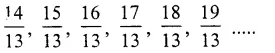Question 5.
Solution: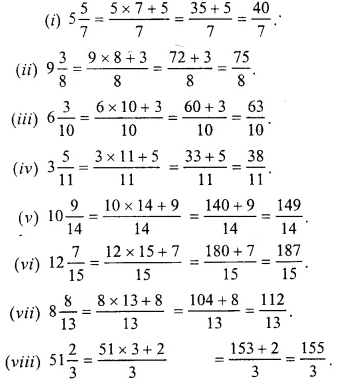Question 6.
Solution: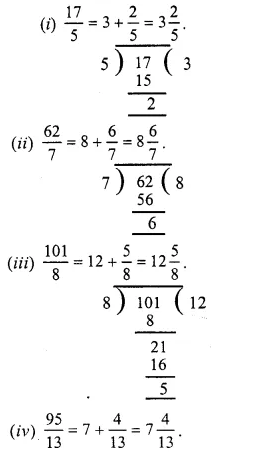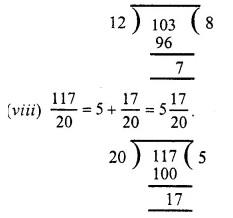Question 7.
Solution: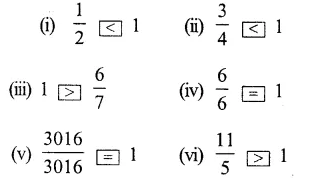Question 8.
Solution:Hope given RS Aggarwal Solutions Class 6 Chapter 5 Fractions Ex 5B are helpful to complete your math homework.

If you have any doubts, please comment below. Learn Insta try to provide online math tutoring for you.